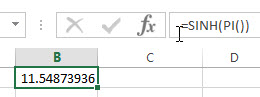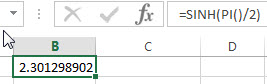# Excel SINH Function

This post will guide you how to use Excel SINH function with syntax and examples in Microsoft excel.

### Description

The Excel SINH function returns the hyperbolic sine of a number.

The SINH function is a build-in function in Microsoft Excel and it is categorized as a Math and Trigonometry Function.

The SINH function is available in Excel 2016, Excel 2013, Excel 2010, Excel 2007, Excel 2003, Excel XP, Excel 2000, Excel 2011 for Mac.

### Syntax

The syntax of the SINH function is as below:

`=SINH (number)`

Where the SINH function argument is:

• number –This is a required argument. Any real number that you want to calculate the hyperbolic sine.

Note:

• If the number argument is not a numeric value, the SINH function will return #VALUE! Error.

### Excel SINH Function Examples

The below examples will show you how to use Excel SINH Function to return the hyperbolic sine of a real number.

1# get the hyperbolic sine of pi radians, enter the following formula in Cell B1.

`=SINH(PI())`2# get the hyperbolic sine of pi/2 radians, enter the following formula in Cell B2.

`=SINH(PI()/2)`### Related Functions

• Excel SIN function
The Excel TANH function returns the hyperbolic tangent of a given number.The syntax of the TANH function is as below:=TANH (number)…
• Excel ASIN function
The Excel ASIN function returns the arcsine value of a number.The syntax of the ASIN function is as below:= ASIN (number)…
• Excel TAN function
The Excel TAN function returns the tangent of a given angle. So you can use this function to calculate the tangent of an angle in radians. The syntax of the TAN function is as below:=TAN (number)…
• Excel TANH function
The Excel TANH function returns the hyperbolic tangent of a given number.The syntax of the TANH function is as below:=TANH (number)…